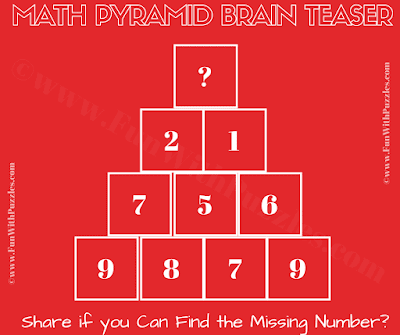## Sunday, November 11, 2018

This Tricky Math Picture Puzzle is for Genius Minds who are good in both Logical Reasoning as well as Mathematics. To solve this Tricky Math Picture Puzzle you will require both your logical and maths skills. In this Tough Math Puzzle, you have to find the value of the missing number at the top of the Pyramid by decoding the relationship among the numbers of this Pyramid. Can you find the relationship between the given numbers and solve this Math Pyramid Brain Teaser?Can you solve this Math Picture Puzzle?

1.i think the answer is 3 , the possible relationship is adding each block and the result is the unit digit.

1.2.1.